## Altitudes and Side Lengths

Before we go around flirting with all the right triangles on the two-dimensional plane (although they are quite saucy), we should get down to business. Get to know them first. Find out what they want and need. Build a solid emotional foundation with them. That kind of stuff is important.

The altitude of a triangle is the distance from one vertex straight down to the opposite side at a 90° angle. It's basically the height of a triangle. Luckily for us, the two legs of a right triangle are its base and height—or altitude. Not too bad.

But what if we lay the triangle down on its longest side? The altitude is the distance from the 90° angle straight down. How do we find the altitude then? Suddenly, things aren't so peachy anymore.

• ### Geometric Mean

To find altitudes of unruly triangles, we can just use the geometric mean, which actually isn't mean at all. It's quite nice. Just multiply two numbers together and take the square root. The positive number is the answer. That's it.

So if you're ever at a bar (drinking a Coca-Cola or chocolate milk, of course) and a right triangle asks you to find the geometric mean of 4 and 16, you won't break a sweat. It'll look like this: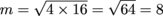Bam. We're done. Now, the reason this is helpful is because it lets the triangle know you care, and it comes from a proportion that looks like this: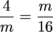If we cross-multiply, we can solve for the geometric mean and it'll give us the definition.

m2 = 4 × 16

Take the square root, and you're back to square one. Easy peasy.

Say that a triangle at the bar, named Aybeesee (or ABC for short), wants you to guess her height. She's a supermodel, so she's really proud of her height.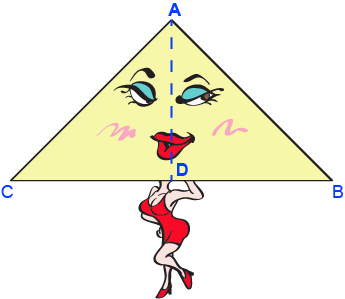Well, her height is the length from point A, where her 90° angle is, down toward her hypotenuse at point D. This line, called the altitude, splits her into two smaller right triangles. Ouch.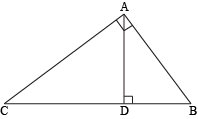Knowing that, there's a possibility that Aybeesee is really two baby triangles in a cocktail dress. Well, maybe not.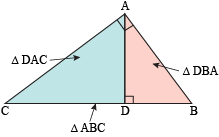They might be different sizes, but these three right triangles are all similar. The "identical angles" kind of similar, not the "Mary-Kate and Ashley Olsen" kind of similar.

If we want to find Aybeesee's height, we can use the similarity of the triangles and make a proportion out of the sides. Aybeesee's height is the short side of one baby triangle and the long side of the other baby triangle.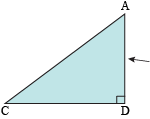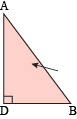Compare the short side to the long side of each triangle and set them equal to each other. It'll look like this: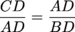Whaddayaknow? It's the same proportion as the geometric mean. So you can find out Aybeesee's height no problem. She'll swoon at your knowledge of geometric means and maybe even pay for your Coca-Cola. Maybe.

Two things to remember about these proportions:

1. The type of side is separated by the fraction.
2. Different triangles are separated by the equal sign.

That means if a hypotenuse is in the numerator of one fraction, another hypotenuse should be on the numerator of the other. If the shortest side of the first triangle is in the denominator, ditto for the second. Basically, this: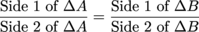The next step is actually solving these bad boys. We're still doing math, so why don't we throw some numbers up in here?

### Sample Problem

What is the length of the altitude of this triangle?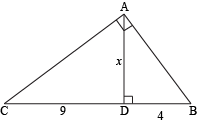If we substitute those numbers into our proportion, we can solve for the length of the altitude. Hopefully it's a smallish number, or else we may get lightheaded.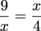Cross-multiplying gives us:

x2 = 9 × 4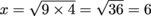We've found the length of the altitude. Not exactly the altitude of Mt. Everest, but it's a start. We can use these proportions to solve for more than just the length of the altitude. If we have the right information, we can find the length of any side of any triangle. Whoa.

### Sample Problem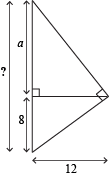Take this triangle, for example. We want to find the length of the biggest triangle's hypotenuse. We already know that 8 is the smaller piece (but we won't tell it because we don't want to hurt its feelings), so all we have to do is find out what the bigger piece is. Setting up our proportion is a good place to start.

The unknown piece, which we'll call a (because it refused to tell us its real name), is the long side of the top triangle, and the altitude is the short side. The altitude is also the long side of the bottom triangle, and the smaller piece of the hypotenuse is the short side. Our proportion should look like this: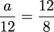12 × 12 = a × 8

144 = 8a

If we divide both sides by 8, we can figure out the mysterious a's real identity: 18. Unfortunately, that's not our answer. We wanted to know the length of the hypotenuse of the biggest triangle, but 18 is only a piece of it. Thankfully, we know the length of the other piece so all that's left is adding them together.

8 + a = 8 + 18 = 26

Done. Feel free to do a victory dance. Don't forget to stretch first, though.

Aside from winning over flirty right triangles at bars, what good are geometric means to us? If you go to museums (and we're sure a cultured student like yourself frequents them), you can use geometric means to look at Van Goghs and Monets.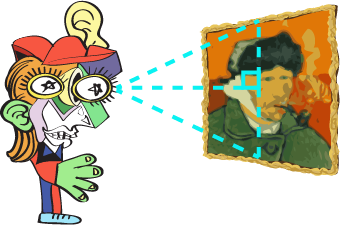When admiring these beautiful (and some not-so-beautiful) works of art, the best distance to stand is the distance of the altitude. That way, you'll be far enough away to see the whole painting without having to move your eyes around but close enough to see the details. Who says math isn't artistic?

• ### The Pythagorean Theorem

Sure, we could cut triangles in two until the cows come home, but there's an even simpler way to calculate the sides of a right triangle.

Mr. Pythagoras has once again come back to haunt us, doing what he's always done: finding distances. Just picture him, in all his ancient wisdom, enlightening us about the wonders of right triangles and how to find their side lengths."I do say, my dear chap. Methinks I've come up with a theorem: if a triangle is a right triangle, the sum of the square of its two legs equals the square of its hypotenuse. Indubitably so."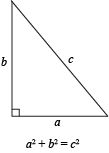What Mr. Pythagoras is trying to say is that for any right triangle, a2 + b2 = c2, where a and b are the lengths of the two sides and c is the hypotenuse. (Don't forget, the hypotenuse is always the side opposite the right angle.) This is called the Pythagorean Theorem.

Mr. Pythagoras is probably telling us the truth. After all, it's hard to doubt a gentleman in a top hat. Just to be sure, though, we should double-check his work and prove his theorem using this triangle.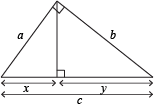We can make two geometric means if we compare the hypotenuse to the long and the short legs of the big triangle.

1. Comparing the hypotenuse to the short leg gives us: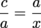2. Comparing the hypotenuse to the long leg gives us: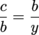If we cross-multiply each of them, we get a2 = cx and b2 = cy. Adding the two equations gives us the first half of Mr. Pythagoras's claim.

a2+ b2 = cx + cy

Almost done. If we factor the right side, we get:

a2+ b2 = c(x + y)

Looking at our original triangle, x + y = c. Plug in the last piece of the puzzle, and we have our final equation.

a2 + b2 = c2

Way to go. Now we can sleep soundly at night, knowing Mr. Pythagoras wasn't bluffing. Or, instead of sleeping, we can use his theorem to figure out some side lengths.

### Sample Problem

What is the length of the hypotenuse of this triangle?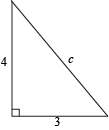We just proved the Pythagorean Theorem. That's a good place to start.

a2 + b2 = c2

The only values we have are the lengths of the legs. We can substitute those in.

(3)2 + (4)2 = c2

Now we'll simplify as much as we can.

9 + 16 = c2
25 = c2

Taking the square root of both sides gives us the answer. Even though we know that square roots have two answers (a positive and a negative answer), lengths can't be negative. That's why we only care about the positive version.

c = 5

We've found the length of the hypotenuse. It's 5, by the way.

The hypotenuse is only one side, though. The other two sides of a right triangle are the legs. That means that there's a two out of three chance that we'll have to calculate the length of one of the legs and not just the hypotenuse.

Don't worry. It's not that much different.

### Sample Problem

What is the length of the missing leg of this triangle?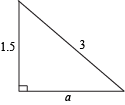Starting with the Pythagorean theorem again, we have a2 + b2 = c2. If we substitute in the lengths of the sides we already know, we'll get a2 + (1.5)2 = (3)2. Let's simplify what we can.

a2 + 2.25 = 9
a ≈ 2.6

We've gotten the hang of it by now. All right triangles follow the Pythagorean Theorem. What happens if we don't know whether or not it's a right triangle? If the three sides satisfy the Pythagorean theorem, then the triangle must be a right triangle. That's called the Converse Pythagorean Theorem.

Easy enough.

A square root sign turns most numbers from nice, whole numbers into long decimals. After Mr. Pythagoras came up with his theorem, he noticed how rare it was for a right triangle to have sides that were only whole numbers.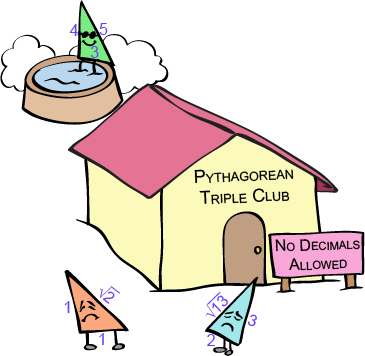He decided set up a special resort called the Pythagorean Triple Club, complete with squash courts and a hot tub. A triangle that's a Pythagorean triple must have sides lengths of three whole numbers and satisfy his a2 + b2 = c2 equation. That means any right triangle with three whole-numbered sides has access to this Pythagorean Triple Club.